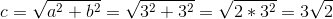# ACT Math : How to find the length of the hypotenuse of a 45/45/90 right isosceles triangle : Pythagorean Theorem

## Example Questions

### Example Question #7 : How To Find The Length Of The Hypotenuse Of A 45/45/90 Right Isosceles Triangle : Pythagorean Theorem

Squarehas a side length of. What is the length of its diagonal?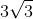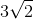Cannot be determined from the information providedExplanation:

The answer can be found two different ways. The first step is to realize that this is really a triangle question, even though it starts with a square. By drawing the square out and adding the diagonal, you can see that you form two right triangles. Furthermore, the diagonal bisects two ninety-degree angles, thereby making the resulting triangles a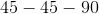triangle.

From here you can go one of two ways: using the Pythagorean Theorem to find the diagonal, or recognizing the triangle as atriangle.

1) Using the Pythagorean Theorem

Once you recognize the right triangle in this question, you can begin to use the Pythagorean Theorem. Remember the formula:, whereandare the lengths of the legs of the triangle, andis the length of the triangle's hypotenuse.

In this case,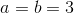. We can substitute these values into the equation and then solve for, the hypotenuse of the triangle and the diagonal of the square: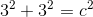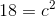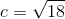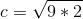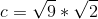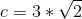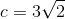The length of the diagonal is.

2) Using Properties ofTriangles

The second approach relies on recognizing atriangle. Although one could solve this rather easily with Pythagorean Theorem, the following method could be faster.triangles have side length ratios of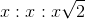, whererepresents the side lengths of the triangle's legs and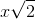represents the length of the hypotenuse.

In this case,because it is the side length of our square and the triangles formed by the square's diagonal.

Therefore, using thetriangle ratios, we havefor the hypotenuse of our triangle, which is also the diagonal of our square.

### Example Question #7 : How To Find The Length Of The Hypotenuse Of A 45/45/90 Right Isosceles Triangle : Pythagorean Theorem

What is the length of the hypotenuse of an isosceles right triangle with an area of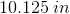?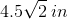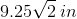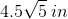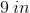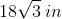Explanation:

Recall that an isosceles right triangle is also atriangle. It has sides that appear as follows: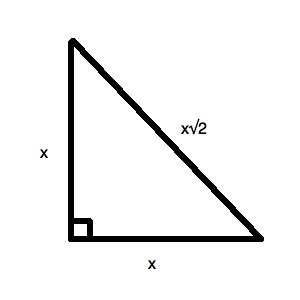Therefore, the area of the triangle is: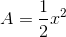, since the base and the height are the same.

For our data, this means: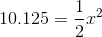Solving for, you get: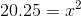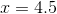So, your triangle looks like this: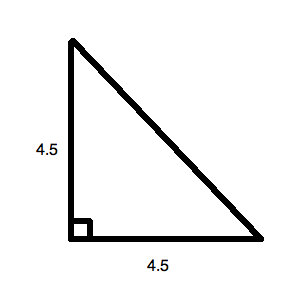Now, you can solve this with a ratio and easily find that it is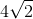.  You also can use the Pythagorean Theorem. To do the latter, it is: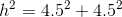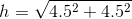Now, just do your math carefully: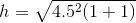That is a weird kind of factoring, but it makes sense if you distribute back into the group. This means you can simplify: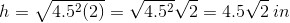### Example Question #8 : How To Find The Length Of The Hypotenuse Of A 45/45/90 Right Isosceles Triangle : Pythagorean Theorem

When the sun shines on a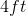pole, it leaves a shadow on the ground that is also. What is the distance from the top of the pole to the end of its shadow?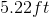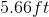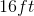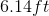Explanation:

The pole and its shadow make a right angle. Because they are the same length, they form an isosceles right triangle (45/45/90). We can use the Pythagorean Theorem to find the hypotenuse.. In this case,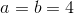. Therefore, we do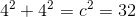. So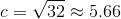### Example Question #211 : Plane Geometry

Find the hypotenuse of an isosceles right triangle given side length of 3.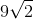Explanation:

To solve, simply use the Pythagorean Theorem.

Recall that an isosceles right triangle has two leg lengths that are equal.

Therefore, to solve for the hypotenuse let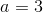and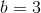in the Pythagorean Theorem.

Thus,